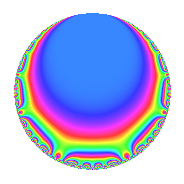# Properties

 Label 4020.2.q.hLevel 4020 Weight 2 Character orbit 4020.q Analytic conductor 32.100 Analytic rank 1 Dimension 2 CM No Inner twists 2

# Related objects

## Newspace parameters

 Level: $$N$$ = $$4020 = 2^{2} \cdot 3 \cdot 5 \cdot 67$$ Weight: $$k$$ = $$2$$ Character orbit: $$[\chi]$$ = 4020.q (of order $$3$$ and degree $$2$$)

## Newform invariants

 Self dual: No Analytic conductor: $$32.0998616126$$ Analytic rank: $$1$$ Dimension: $$2$$ Coefficient field: $$\Q(\sqrt{-3})$$ Coefficient ring: $$\Z[a_1, \ldots, a_{7}]$$ Coefficient ring index: $$1$$ Sato-Tate group: $\mathrm{SU}(2)[C_{3}]$

## $q$-expansion

Coefficients of the $$q$$-expansion are expressed in terms of a primitive root of unity $$\zeta_{6}$$. We also show the integral $$q$$-expansion of the trace form.

 $$f(q)$$ $$=$$ $$q + q^{3} - q^{5} + \zeta_{6} q^{7} + q^{9} +O(q^{10})$$ $$q + q^{3} - q^{5} + \zeta_{6} q^{7} + q^{9} -3 \zeta_{6} q^{11} + ( -5 + 5 \zeta_{6} ) q^{13} - q^{15} + ( -3 + 3 \zeta_{6} ) q^{17} + ( 1 - \zeta_{6} ) q^{19} + \zeta_{6} q^{21} + ( -3 + 3 \zeta_{6} ) q^{23} + q^{25} + q^{27} + 3 \zeta_{6} q^{29} -5 \zeta_{6} q^{31} -3 \zeta_{6} q^{33} -\zeta_{6} q^{35} + ( 7 - 7 \zeta_{6} ) q^{37} + ( -5 + 5 \zeta_{6} ) q^{39} -9 \zeta_{6} q^{41} -4 q^{43} - q^{45} + 3 \zeta_{6} q^{47} + ( 6 - 6 \zeta_{6} ) q^{49} + ( -3 + 3 \zeta_{6} ) q^{51} -6 q^{53} + 3 \zeta_{6} q^{55} + ( 1 - \zeta_{6} ) q^{57} -12 q^{59} + ( 1 - \zeta_{6} ) q^{61} + \zeta_{6} q^{63} + ( 5 - 5 \zeta_{6} ) q^{65} + ( -7 - 2 \zeta_{6} ) q^{67} + ( -3 + 3 \zeta_{6} ) q^{69} -3 \zeta_{6} q^{71} + ( 7 - 7 \zeta_{6} ) q^{73} + q^{75} + ( 3 - 3 \zeta_{6} ) q^{77} -17 \zeta_{6} q^{79} + q^{81} + ( -15 + 15 \zeta_{6} ) q^{83} + ( 3 - 3 \zeta_{6} ) q^{85} + 3 \zeta_{6} q^{87} -6 q^{89} -5 q^{91} -5 \zeta_{6} q^{93} + ( -1 + \zeta_{6} ) q^{95} + ( -17 + 17 \zeta_{6} ) q^{97} -3 \zeta_{6} q^{99} +O(q^{100})$$ $$\operatorname{Tr}(f)(q)$$ $$=$$ $$2q + 2q^{3} - 2q^{5} + q^{7} + 2q^{9} + O(q^{10})$$ $$2q + 2q^{3} - 2q^{5} + q^{7} + 2q^{9} - 3q^{11} - 5q^{13} - 2q^{15} - 3q^{17} + q^{19} + q^{21} - 3q^{23} + 2q^{25} + 2q^{27} + 3q^{29} - 5q^{31} - 3q^{33} - q^{35} + 7q^{37} - 5q^{39} - 9q^{41} - 8q^{43} - 2q^{45} + 3q^{47} + 6q^{49} - 3q^{51} - 12q^{53} + 3q^{55} + q^{57} - 24q^{59} + q^{61} + q^{63} + 5q^{65} - 16q^{67} - 3q^{69} - 3q^{71} + 7q^{73} + 2q^{75} + 3q^{77} - 17q^{79} + 2q^{81} - 15q^{83} + 3q^{85} + 3q^{87} - 12q^{89} - 10q^{91} - 5q^{93} - q^{95} - 17q^{97} - 3q^{99} + O(q^{100})$$

## Character Values

We give the values of $$\chi$$ on generators for $$\left(\mathbb{Z}/4020\mathbb{Z}\right)^\times$$.

 $$n$$ $$1141$$ $$2011$$ $$2681$$ $$3217$$ $$\chi(n)$$ $$-\zeta_{6}$$ $$1$$ $$1$$ $$1$$

## Embeddings

For each embedding $$\iota_m$$ of the coefficient field, the values $$\iota_m(a_n)$$ are shown below.

For more information on an embedded modular form you can click on its label.

Label $$\iota_m(\nu)$$ $$a_{2}$$ $$a_{3}$$ $$a_{4}$$ $$a_{5}$$ $$a_{6}$$ $$a_{7}$$ $$a_{8}$$ $$a_{9}$$ $$a_{10}$$
841.1
 0.5 − 0.866025i 0.5 + 0.866025i
0 1.00000 0 −1.00000 0 0.500000 0.866025i 0 1.00000 0
3781.1 0 1.00000 0 −1.00000 0 0.500000 + 0.866025i 0 1.00000 0
 $$n$$: e.g. 2-40 or 990-1000 Significant digits: Format: Complex embeddings Normalized embeddings Satake parameters Satake angles

## Inner twists

Char. orbit Parity Mult. Self Twist Proved
1.a Even 1 trivial yes
67.c Even 1 yes

## Hecke kernels

This newform can be constructed as the intersection of the kernels of the following linear operators acting on $$S_{2}^{\mathrm{new}}(4020, [\chi])$$:

 $$T_{7}^{2} - T_{7} + 1$$ $$T_{11}^{2} + 3 T_{11} + 9$$ $$T_{17}^{2} + 3 T_{17} + 9$$